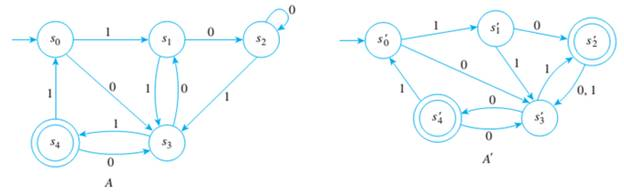Chapter 12.3, Problem 10ES### Discrete Mathematics With Applicat...

5th Edition
EPP + 1 other
ISBN: 9781337694193

#### Solutions

Chapter
Section### Discrete Mathematics With Applicat...

5th Edition
EPP + 1 other
ISBN: 9781337694193
Textbook Problem
1 views

# Are the automata A and A’ shown below equivalent?To determine

To check whether the given two finite-state automata A and A' are equivalent or not.

Explanation

Given information:

Two finite-state automatons are given as follows.

Formula used:

Two finite-state automata are said to be equivalent if, and only if, the accepted languages of two automata should be equal to each other.

Let A and A' be two finite-state automata defined on the same set of input symbols I. Then A is equivalent to A' if, and only if, L(A)=L(A').

For both finite-state automatons, the set I contain only 0 and 1 as the inputs. To become these two automatons equivalent to each other, the accepted languages should be equal.

If the accepted language of A (L(A)) is equal to the accepted language of A' (L(A')), for each input string belongs to L(A), there exists an input string in L(A') that equal to the first string

### Still sussing out bartleby?

Check out a sample textbook solution.

See a sample solution

#### The Solution to Your Study Problems

Bartleby provides explanations to thousands of textbook problems written by our experts, many with advanced degrees!

Get Started